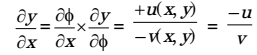## Fluid Mechanics and Hydraulic Machinery Miscellaneous

#### Fluid Mechanics and Hydraulic Machinery

1. If the fluid velocity for a potential flow is given by V(x, y) = u(x, y)i + v(x, y)j with usual notations, then the slope of the potential line at (x, y) is

1. Here V(x, y) = u(x, y) i + v(x, y) j

 As we know that u = -δφ δx

 ∴ -δφ = -v(x,y) ....(i) δx

 Similarly v = -δφ δy

 ∴ -δφ = -v(x,y) ....(ii) δy

From equations (i) and (ii) we get,##### Correct Option: B

Here V(x, y) = u(x, y) i + v(x, y) j

 As we know that u = -δφ δx

 ∴ -δφ = -v(x,y) ....(i) δx

 Similarly v = -δφ δy

 ∴ -δφ = -v(x,y) ....(ii) δy

From equations (i) and (ii) we get,1. Consider a velocity field V K(yi + xk), where K is a constant. The vorticity, Ω Z, is

1. NA

##### Correct Option: A

NA

1. Consider the following statements regarding streamline(s):
(i) It is a continuous line such that the tangent at any point on it shows the velocity vector at that point
(ii) There is no flow across streamlines
(iii)
 dx = dy = dz is the differential equation of a streamline, where u, v and w are velocities in directions x, y and z, respectively u v w

(iv) In an unsteady flow, the path of a particle is a streamline
Which one of the following combinations of the statements is true?

1. NA

##### Correct Option: D

NA

1. A cube of side 100 mm is placed at the bottom of an empty container on one of its faces. The density of the material of the cube is 800 kg/m3. Liquid of densit y 1000 k g/m3 is now poured into the container. The minimum height to which the liquid needs to be poured into the container for the cube to just lift up is _____ mm.

1. Given, side of cube (a) = 100 m
Density of material of cube = 800 kg/m3
Poured liquid density = 1000 kg/m3
Now, Minimum height to which the liquid needs to be poured into the container for the cube to just lift up, should satisfy the condition
FB ≥ (mg)block
ρfgVfd ≥ (ρV)block× g
1000 × 0.1 × h ≥ 800 × 0.1 × 0.1 × 0.1
h ≥ 0.08 m
h ≥ 80 m

##### Correct Option: A

Given, side of cube (a) = 100 m
Density of material of cube = 800 kg/m3
Poured liquid density = 1000 kg/m3
Now, Minimum height to which the liquid needs to be poured into the container for the cube to just lift up, should satisfy the condition
FB ≥ (mg)block
ρfgVfd ≥ (ρV)block× g
1000 × 0.1 × h ≥ 800 × 0.1 × 0.1 × 0.1
h ≥ 0.08 m
h ≥ 80 m

1. For the stability of a floating body the

1. NA

NA